# Density

Density (symbol: ρ - Greek: rho) is a measure of mass per volume. The average density of an object equals its total mass divided by its total volume. An object made from a comparatively dense material (such as iron) will have less volume than an object of equal mass made from some less dense substance (such as water).

## Contents

The SI unit of density is the kilogram per cubic metre (kg/m3)

## Definition

Under specified conditions of temperature and pressure, the density of a fluid is defined as described below. However, the density of a solid material can be different, depending on exactly how it is defined. Take sand for example. If you gently fill a container with sand, and divide the mass of sand by the container volume you get a value termed loose bulk density. If you took this same container and tapped on it repeatedly, allowing the sand to settle and pack together, and then calculate the results, you get a value termed tapped or packed bulk density. Tapped bulk density is always greater than or equal to loose bulk density. In both types of bulk density, some of the volume is taken up by the spaces between the grains of sand.

Also, in terms of candy making, density is affected by the melting and cooling processes. Loose granular sugar, like sand, contains a lot of air and is not tightly packed, but when it has melted and starts to boil, the sugar loses its granularity and entrained air and becomes a fluid. When you mold it to make a smaller, compacted shape, the syrup tightens up and loses more air. As it cools, it contracts and gains moisture, making the already heavy candy even more dense.$\rho = \frac{m}{V}$

where

ρ is the object's density (measured in kilograms per cubic metre)
m is the object's total mass (measured in kilograms)
V is the object's total volume (measured in cubic metres)

A more theoretical definition is also available. Density can be calculated based on crystallographic information and molar mass: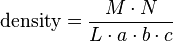$\mbox{density} = \frac{M \cdot N} {L \cdot a \cdot b \cdot c}$

where

M is molar mass
N is the number of atoms in a unit cell
L is Loschmidt or Avogadro's number
a, b, c are the lattice parameters

The density with respect to temperature, T, has the following relation: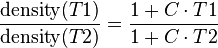$\frac{\mbox{density}(T1)} {\mbox{density}(T2)} = \frac{1 + C \cdot T1} {1 + C \cdot T2}$

where

C is the coefficient of cubic expansion.

Experimentally density can be found by measuring the dry weight (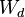$W_d$ ), the wet weight (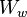$W_w$) and submersed weight (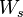$W_s$), usually in water.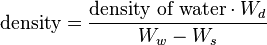$\mbox{density} = \frac{\mbox{density of water} \cdot W_d} {W_w - W_s}$

## Other units

Density in terms of the SI base units is expressed in kilograms per cubic meter (kg/m3). Other units fully within the SI include grams per cubic centimeter (g/cm3) and megagrams per cubic metre (Mg/m3). Since both the litre and the tonne or metric ton are also acceptable for use with the SI, a wide variety of units such as kilograms per litre (kg/L) are also used. Imperial units or U.S. customary units, the units of density include pounds per cubic foot (lb/ft³), pounds per cubic yard (lb/yd³), pounds per cubic inch (lb/in³), ounces per cubic inch (oz/in³), pounds per gallon (for U.S. or imperial gallons) (lb/gal), pounds per U.S. bushel (lb/bu), in some engineering calculations slugs per cubic foot, and other less common units.

The maximum density of pure water at a pressure of one standard atmosphere is 999.861kg/m3; this occurs at a temperature of about 3.98 °C (277.13 K).

From 1901 to 1964, a litre was defined as exactly the volume of 1 kg of water at maximum density, and the maximum density of pure water was 1.000 000 kg/L (now 0.999 972 kg/L). However, while that definition of the litre was in effect, just as it is now, the maximum density of pure water was 0.999 972 kg/dm3. During that period students had to learn the esoteric fact that a cubic centimeter and a milliliter were slightly different volumes, with 1 mL = 1.000 028 cm³. (Often stated as 1.000 027 cm³ in earlier literature).

Density will determine the "order" in which each substance will appear in a bottle. For example, if substance A has a density of .64g/cm3, and Substance B has a density of .84g/cm3, Substance A will be above Substance B in a container due to the simple fact that its density is lower. One example of this is oil and water, where the oil will remain above.

## Measurement of Density

A common device for measuring fluid density is a pycnometer. A device for measuring absolute density of a solid is a gas pycnometer.

For a rectagular solid, the formula Mass / (Length x Width x Height) can be used. For an irregularly shaped solid, Displacement (fluid) can be used in place of L x W x H.

## Relative Density

Relative density (known as specific gravity when water is the referent) is a measure of the density of a material. It is dimensionless, equal to the density of the material divided by some reference density (most often the density of water, but sometimes the air when comparing to gases):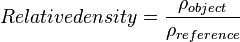$Relative density = \frac{\rho_{object}}{\rho_{reference}}$

where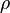$\rho$ denotes density.

Since water's density is 1.0 × 103 kg/m3 in SI units, the relative density of a material is approximately the density of the material measured in kg/m3 divided by 1000 (the density of water). There are no units of measurement.

Water's density can also be measured as nearly one gram per cubic centimeter (at maximum density) in non-SI units. The relative density therefore has nearly the same value as density of the material expressed in grams per cubic centimeter, but without any units of measurement.

Relative density or specific gravity is often an ambiguous term. This quantity is often stated for a certain temperature. Sometimes when this is done, it is a comparison of the density of the commodity being measured at that temperature, with the density of water at the same temperature. But they are also often compared to water at a different temperature.

Relative density is often expressed in forms similar to this:

relative density: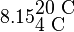$8.15_{\mbox{4 C}}^{\mbox{20 C}} \,\,$ or specific gravity: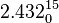$2.432_0^{15}$

The superscripts indicate the temperature at which the density of the material is measured, and the subscripts indicate the temperature of the water to which it is compared.

Density of water

Density of water at 1 atm (101.325 kPa, 14.7 psi)
Temperature Density
Celsius Fahrenheit kg/m3
0 °C 32 °F 999.8425
4.0 °C 39.2 °F 999.9750
15 °C 59 °F 999.1026
20 °C 68 °F 998.2071
25 °C 77 °F 998.0479
37.0 °C 98.6 °F 993.3316
100 °C 212 °F 958.3665

Water is nearly incompressible. But it does compress a little; it takes pressures over about 400 kPa or 4 atmospheres before water can reach a density of 1,000.000 kg/m3 at any temperature.

Relative density is often used by geologists and mineralogists to help determine the mineral content of a rock or other sample. Gemologists use it as an aid in the identification of gemstones. The reason that relative density is measured in terms of the density of water is because that is the easiest way to measure it in the field. Basically, density is defined as the mass of a sample divided by its volume. With an irregularly shaped rock, the volume can be very difficult to accurately measure. One way is to put it in a water-filled graduated cylinder and see how much water it displaces. Relative density is more easily and perhaps more accurately measured without measuring volume. Simply suspend the sample from a spring scale and weigh it under water. The following formula for measuring specific gravity: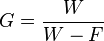$G = \frac{W}{W - F}$

where

G is the relative density,
W is the weight of the sample (measured in pounds-force, newtons, or some other unit of force),
F is the force, measured in the same units, while the sample was submerged.

Note that with this technique it is difficult to measure relative densities less than one, because in order to do so, the sign of F must change, requiring the measurement of the downward force needed to keep the sample underwater.

Another practical method uses three measurements. The mineral sample is weighed dry. Then a container filled to the brim with water is weighed, and weighed again with the sample immersed, after the displaced water has overflowed and been removed. Subtracting the last reading from the sum of the first two readings gives the weight of the displaced water. The relative density result is the dry sample weight divided by that of the displaced water. This method works with scales that can't easily accommodate a suspended sample, and also allows for measurement of samples that are less dense than water. Surface tension of the water may keep a significant amount of water from overflowing, which is especially problematic for small objects being immersed. A workaround would be to use a water container with as small a mouth as possible.

### Specific Gravity of water

The specific gravity is defined as the ratio of specific weight of the material to the specific weight of distilled water. (S = specific weight of the material/specific weight of water). This implies that if the specific gravity is approximately equal to 1.000, then the specific weight of the material is close to the specific weight of water. If the specific gravity is large this means that the specific weight of the material is much larger than the specific weight of water and if the specific gravity is small this implies that the specific weight of the material is much smaller than the specific weight of water. The specific weight of a gas is generally defined by comparing the specific gravity of air at a temperature of 20 degrees Celsius and a pressure of 101.325 kPa absolute, where the density is 1.205 kg/m3. Specific Gravity is unitless.

Specific gravity of Biogas== The density of biogas at 50% methane proportion is 1.227 kg/m3. Hence Specific gravity of Biogas is 1.227.

The kidneys and specific gravity==

The role of the kidneys in the human is to aid the body in its riddance of bodily toxins. The body effectively excretes these toxins via urination, and the role of the kidney is to concentrate as many toxins as it can into the least amount of urine to provide for a more efficient emission. The specific gravity of urine is the measurement of density of these minerals and toxins in the urine in relation to the density of the water; basically, specific gravity is measuring the concentration of solutes in the solution.

The body generates countless toxins every moment. In the kidneys, these toxins are dissolved in water so the body can filter them out through urination. A healthy kidney will use fewer fluids to eliminate these toxins to promote fluid concentration. In an unhealthy kidney, however, more water might be required to dissolve these toxins.

Such is the case in a person with renal failure. A person with this problem would drink more water to account for the excess water loss and his specific gravity would be lower. If the kidneys fail over an extended period of time, more water would be needed in order to concentrate the same amount of urine. Toxin levels in the body would rise, and ultimately, one could not keep up with the amount of water necessary to excrete the toxins. The rising toxin levels in the body do not increase the specific gravity in the urine because these toxins are not manifesting themselves in the urine which is still heavily diluted. The urine will have the same fixed gravity regardless of water intake.

Lowered specific gravity can also occur in diabetics that are lacking an anti-diuretic hormone. This hormone generally sends an appropriate amount of fluids into the bloodstream, and less water is available for urination. A lack of ADH would increase the water volume in the kidneys. A person with this issue could urinate up to fifteen or twenty liters a day with a low specific gravity. Another occurrence resulting in low specific gravity is when the kidney tubules are damaged and can no longer absorb water. Such an instance would also result in a higher water volume in urine.

A high specific gravity is most often indicative of dehydration. If a person has gone without water for a day, his water level in his blood is lowered, and his brain signals the release of an anti-diuretic hormone which redirects water from urine into the bloodstream. Naturally, a lesser volume of liquid provided for urination with the same amount of toxins would result in a higher specific gravity—a higher density of the solutes. There are also other instances where the specific gravity might be raised. When the renal blood pressure is lowered, the artery must compensate with other fluids. Water is reabsorbed into the bloodstream to balance out the volume of blood and the volume of water in urine is subsequently lowered. As water is also used to control body temperature, when the body temperature goes up, less water is in the kidneys as it is used to aid in perspiration.

When testing for specific gravity, one should be aware that enzymes or dyes used in diagnostic tests can increase specific gravity. A pattern presented throughout the report indicates that when urine volume is increased, the specific gravity is lowered. This can be logically understood upon the cognitive awareness that when there is an identical amount of a solute in two solutions, the solution with a greater liquid will be less dense that that of the lesser liquid. As stated before, specific gravity measures the concentration levels of the solute in the solution, ergo the solution of greater volume has a lower specific gravity.

## Density of substances

Perhaps the highest density known is reached in neutron star matter (neutronium). The singularity at the centre of a black hole, according to general relativity, does not have any volume, so its density is undefined.

The densest naturally occurring substance on Earth appears to be iridium, at about 22650 kg/m3. However, because this calculation requires a strong theoretical basis, and the difference between iridium and osmium is so small, definitively stating one or the other is more dense is not possible at this time.

A table of masses of various substances:

 Substance Density in kg/m3 Particles per cubic metre Iridium 22650 1.06 ×1029 Osmium 22610 7.16 ×1028 Platinum 21450 6.62 ×1028 Gold (0°C) 19300 5.90 ×1028 Tungsten 19250 6.31 ×1028 Uranium 19050 4.82 ×1028 Mercury 13580 4.08 ×1028 Palladium 12023 6.8 ×1028 Lead 11340 3.3 ×1028 Silver 10490 5.86 ×1028 Copper 8960 8.49 ×1028 Iron 7870 8.49 ×1028 Steel 7850 Tin 7310 3.71 ×1028 Titanium 4507 5.67 ×1028 Diamond 3500 1.75 ×1029 Basalt 3000 Granite 2700 Aluminium 2700 6.03 ×1028 Graphite 2200 1.10 ×1029 Magnesium 1740 4.31 ×1028 PVC 1300 Seawater (15°C) 1025 Water (25 °C) 998 3.34 ×1028 Ice (0°C) 917 3.07 ×1028 Polyethylene 910 Ethyl alcohol 790 1.03 ×1028 Gasoline 730 Liquid Hydrogen 68 4.06 ×1028 Aerogel 3 any gas 0.0446 times the average molecular mass (in g/mol), hence between 0.09 and ca. 13.1 (at 0°C and 1 atm) For example air (0°), (25°) 1.29, 1.17
 Density of air ρ vs. temperature °C T in °C ρ in kg/m3 - 10 1.341 - 5 1.316 0 1.293 + 5 1.269 + 10 1.247 + 15 1.225 + 20 1.204 + 25 1.184 + 30 1.164

Note the low density of aluminium compared to most other metals. For this reason, aircraft are made of aluminium. Also note that air has a nonzero, albeit small, density. Aerogel is the world's lightest solid.